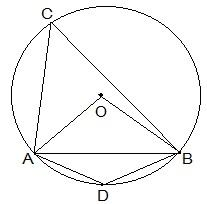Guru

# A chord of a circle is equal to the radius of the circle. Find the angle subtended by the chord at a point on the minor arc and also at a point on the major arc. Q.2

• 0

I want to know the best answer of the question from Circles chapter of class 9th ncert math. The question from exercise 10.5of math. Give me the easy way for solving this question of  2 A chord of a circle is equal to the radius of the circle. Find the angle subtended by the chord at a point on the minor arc and also at a point on the major arc.

Share

1.Here, the chord AB is equal to the radius of the circle. In the above diagram, OA and OB are the two radii of the circle.

Now, consider the ΔOAB. Here,

AB = OA = OB = radius of the circle.

So, it can be said that ΔOAB has all equal sides and thus, it is an equilateral triangle.

∴ AOC = 60°

And, ACB = ½ AOB

So, ACB = ½ × 60° = 30°

Now, since ACBD is a cyclic quadrilateral,

ADB +ACB = 180° (Since they are the opposite angles of a cyclic quadrilateral)

So, ADB = 180°-30° = 150°

So, the angle subtended by the chord at a point on the minor arc and also at a point on the major arc are 150° and 30° respectively.

• 0